# Pre-Algebra : Negative Numbers

## Example Questions

### Example Question #21 : Negative Numbers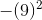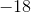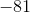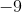Explanation:

Let's focus on the exponent.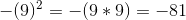. Remember a value raised to something means that value is multiplied by that power. The negative is applied after the math is done.

### Example Question #21 : Negative Numbers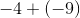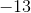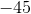Explanation:

When we add a negative number, the sign turns negative. Since we are adding two negative numbers, we treat this as an addition problem and add a minus sign in the end.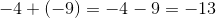### Example Question #1 : Multiply And Divide Rational Numbers Using Properties Of Operations: Ccss.Math.Content.7.Ns.A.2c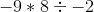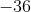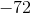Explanation:

Let's work from left to right since it's only multiplication and division. Since there is only one negative number, the product of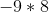is negative which is. Now we have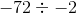. Two negative numbers in a  division problem makes the answer positive.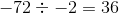### Example Question #1 : Understand Distances Between Numbers On A Number Line: Ccss.Math.Content.7.Ns.A.1b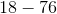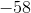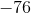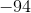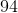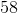Explanation:

When dealing with negative numbers, let's see which number is greater.is greater thanand is negative so the answer is negative. We treat this as a subtraction problem.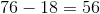. Because our answer should be negative, the correct answer is### Example Question #2 : Solve Problems With The Four Operations With Rational Numbers: Ccss.Math.Content.7.Ns.A.3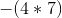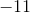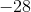Explanation:

We need to take care of the parentheses first because of PEMDAS. Parentheses has priority over everything. The product is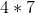is. Because there is a negative sign outside the parentheses, we need to add it to our answer which now becomes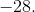### Example Question #321 : The Number System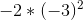Explanation:

Remember PEMDAS. Parentheses comes first then multiplication.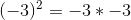. We are multiplying two negatives which make a positive number, in this case. Next, we have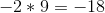since there is only one negative number, the answer is negative.

### Example Question #241 : Operations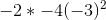Explanation:

Remember PEMDAS. Parenthesis comes first then multiplication.. We are multiplying two negatives which make a positive number, in this case. Next, we have just multiplication of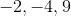. Since there are two negative numbers and one positive number being multiplied, the answer is positive.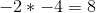and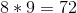### Example Question #1 : Understand Distances Between Numbers On A Number Line: Ccss.Math.Content.7.Ns.A.1b

Solve: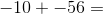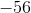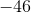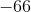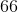Explanation:

Remember that adding a negative number is the same as subtracting that same number if it were positive.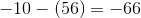### Example Question #22 : Negative Numbers

Solve: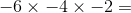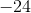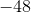Explanation:

When you multiply a negative by another negative, the answer will be positive. If you multiply a third negative number, the answer becomes negative again. The rule is:

1. multiplication of an EVEN number of negative numbers = positive number

2. multiplication of an ODD number of a negative numbers = negative number

Therefore,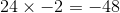### Example Question #1 : Subtract Rational Numbers And Understand The Absolute Value Of Their Difference: Ccss.Math.Content.7.Ns.A.1c

Solve: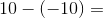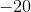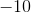Explanation:

Remember that subtracting a negative number is just like adding the positive of that number, because you move to the right (more positive) on the number line. Therefore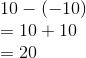### All Pre-Algebra Resources